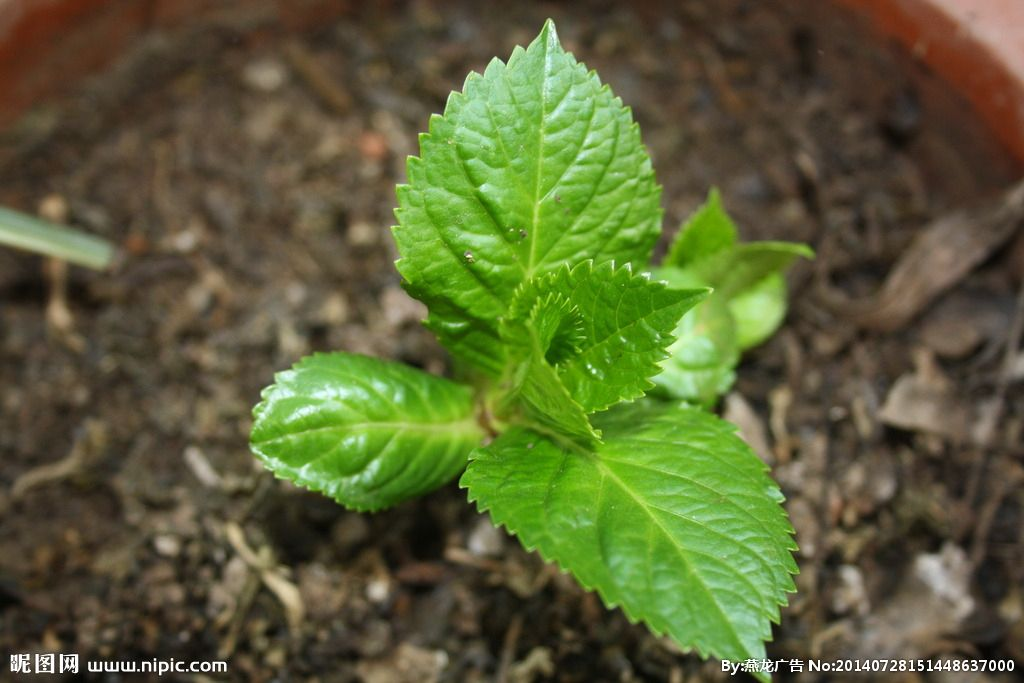# A scheme for simulating sustainable leaf area index based on mass-balance equationIt has been confirmed that the universal productivity model proposed by Wang et al. (named Pmodel) can well simulate the gross primary productivity (GPP) on the monthly scale. However, if we want to drive this model, we need the information of plant canopy coverage, such as leaf area index (LAI), as the input. This model can not be used to predict the future GPP because of the dependence for observation of canopy coverage at present. Now, we are working on proposing a scheme starting from the first-principles, such as mass-balance and optimality, to predict the dynamic of LAI. Then we incorporate this LAI scheme into Pmodel, and the diagnostic Pmodel is developed into the prognostic Pmodel. Here, we attempt to project a scheme starting from carbon mass-balance.

Plant leaves are the basic organs for plant photosynthetic. The amount of plant leaves reflected by the leaf area index is closely relative to the productivity of plant canopy. One the one hand, the LAI influences the fraction of radiation intercepted by canopy, then the production of plant canopy. On the other hand, the LAI is also limited by the plant productivity (GPP), which determines how many potential product of assimilation that could be allocated to leaf. Plants have adaptive strategy to their environment. This adaptive strategy ensures that the carbon supply for building leaf can match with the demand. So, the two process can be dynamically balanced under the canopy closure condition, and plants keep the sustainable LAI for a certain time. Here we can get the value of sustainable LAI by resolving the closed equations as follows:

LMA × LAIs = ∑GPP × h                            (1a)

∑GPP = LUE × ∑PAR × fAPARm                           (1b)

fAPARm = δ × [1 – exp(–k LAIs)]                          (1c)

where LMA is the leaf mass per area; LAIs is the sustainable leaf area index; h is the fraction of GPP allocated to leaf mass; ∑GPP is the accumulated GPP from growing start until canopy closure; LUE is the light use efficiency; ∑PAR is the accumulated photosynthetically active radiation (PAR) from growing start until canopy closure; fAPARm is the mean fraction of PAR intercepted by canopy; δ is the ratio of the mean fraction of absorbed PAR to maximum fraction of absorbed PAR according to the Beer’s law; k = 0.5 is the light extinction coefficient.

Let a be an intermediate variable as follows:

a = h × LUE × ∑PAR × δ/LMA                                  (2)

Substitute a into equations 1a, 1b and 1c, we get a simplified equation as follows:

LAIs = a × [1 – exp(–k LAIs)]                                       (3)

We can get the analytical resolution of equation 3 using the Lambert W function as follow:

LAIs = a + 1/k W0[−k a exp (−k a)]                              (4)

Where Lambert W function is the inverse of the f(x) =x exp(x). Solutions of the Lambert function have already been embedded in some software, such as R package ‘lamW’.

In this case, if we know the value of a and k (k = 0.5), we can get the simulation of sustainable LAI under corresponding to environment.

We can calculate LUE based on environmental variables using Pmodel as follows:

LUE = F0 × m × √[1 – (c*/m)2/3]                                    (5a)

m = (caG*)/{ca + 2G* + 3G*[1.6h*D / b(K + G)]}                   (5b)

Where Φ0 is the intrinsic quantum yield (mol CO2 mol−1); ca is the ambient atmospheric CO2 partial pressure (Pa); Γ* is the photorespiratory compensation point (Pa); η* is the viscosity of water, relative to its value at 25 ˚C; D is the vapour pressure deficit (Pa); Kis the effective Michaelis-Menten coefficient of Rubisco (Pa); c* = 0.41 and β = 146 are dimensionless constants.

The condition of water supply can influence the value of h, so h can be wrote as a function of moisture index (a) as follows:

h = f (a)                                                         (6)

Where we fitted linear regression according to observation, and get the empirical function of equation 6, h = −0.11a + 0.29. a is the moisture index, which represents the ratio of actual evapotranspiration to equilibrated evapotranspiration.

Figure 1 is a test about the response of sustainable LAI to different environmental factors.

Figure 1 The response of sustainable LAI to different environmental factors. We set a baseline condition where temperature is 15℃; accumulated photosynthetically active radiation is 1500 (mol photon m−2); CO2 concentration is 400 ppm; vapor pressure deficit 1 kPa; moisture index is 1.26 and air pressure is 101 kPa, then changed one of the environment factors at a time to test the response.

References:

Qiao, S. et al., 2020. Extending a first-principles primary production model to predict wheat yields. Agricultural and Forest Meteorology 287.

Wang, H. et al., 2017. Towards a universal model for carbon dioxide uptake by plants. Nat Plants, 3(9): 734-741.

Xin, Q. et al., 2020. A Semiprognostic Phenology Model for Simulating Multidecadal Dynamics of Global Vegetation Leaf Area Index. Journal of Advances in Modeling Earth Systems 12(7).

Close